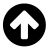# JavaScript Terms

## Event Triggers

 onabort => The user aborted loading the page onblur => The user left the object onchange => The user changed the object onclick => The user clicked the object onerror => The script encountered an error onfocus => The user made an object active onload => The object finished loading onmouseover => The cursor moved over an object onmouseout => The cursor moved off an object onselect => The user selected the contents of an object onsubmit => The user submitted a form onunload => The user left the page

## Comparisons

 X == Y => Returns True if X equals Y X === Y => Returns True if X is identical to Y X != Y => Returns True if X does not equal Y X !== Y => Returns True if X is not identical to Y

## Arithmetic Operations

 + => Addtion - The addition operator (+) adds a value: - => Subtraction - The subtraction operator (-) subtracts a value. * => Multiplication - The multiplication operator (*) multiplies a value. / => Division - The division operator (/) divides a value. % => Modulud - The modular operator (%) returns division remainder. ++ => Increment - The increment operator (++) increments a value. -- => Decrement - The decrement operator (--) decrements a value.

## Assignment Operations

 = => The = assignment operator assigns a value to a variable. += => The += assignment operator adds a value to a variable. -= => The -= assignment operator subtracts a value from a variable. *= => The *= assignment operator multiplies a variable. /= => The /= assignment divides a variable. %= => The %= assignment operator assigns a remainder to a variable.

## IF statements

This can also be written in short hand

This is a common do have several different things happen depending on your conditions. Below is an example that shows how you can use this to change a variable.

This can also be written in short hand

## Switch / Case statements

The switch and case statement is useful when you want to set up several options instead of just two. This can still be done with an if statement if you nest several of them inside of each other. This technique can be easier to read, write, and use as well as providing a catch all that is pretty easy.

This is in my opinion a very useful when you have several options that are possible and a default answer as well.

## Try / Catch statements

This is good for catching errors.